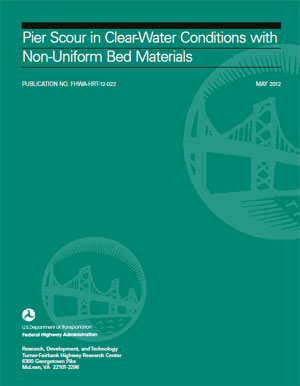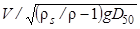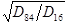U.S. Department of Transportation
1200 New Jersey Avenue, SE
Washington, DC 20590
202-366-4000

Federal Highway Administration Research and Technology
Coordinating, Developing, and Delivering Highway Transportation Innovations

 REPORT This report is an archived publication and may contain dated technical, contact, and link information
 Publication Number:  FHWA-HRT-12-022    Date:  May 2012
 Publication Number: FHWA-HRT-12-022 Date: May 2012

# Pier Scour in Clear-Water Conditions With Non-Uniform Bed Materials

PDF files can be viewed with the Acrobat® Reader®## FOREWORD

The study described in this report was conducted at the Federal Highway Administration's Turner-Fairbank Highway Research Center (TFHRC) J. Sterling Jones Hydraulics Laboratory in response to State transportation departments' requests for new design guidance to predict bridge pier scour for coarse bed material. The study included experiments at the TFHRC J. Sterling Jones Hydraulics Laboratory and analysis of data from the Colorado State University and the United States Geological Survey. This report will be of interest to hydraulic engineers and bridge engineers involved in bridge foundation design. It is being distributed as an electronic document through the TFHRC Web site (https://www.fhwa.dot.gov/research/)

Jorge E. Pagan-Ortiz

Director, Office of Infrastructure
Research and Development

### Notice

This document is disseminated under the sponsorship of the U.S. Department of Transportation in the interest of information exchange. The U.S. Government assumes no liability for the use of the information contained in this document. This report does not constitute a standard, specification, or regulation.

The U.S. Government does not endorse products or manufacturers. Trademarks or manufacturers' names appear in this report only because they are considered essential to the objective of the document.

### Quality Assurance Statement

The Federal Highway Administration (FHWA) provides high-quality information to serve Government, industry, and the public in a manner that promotes public understanding. Standards and policies are used to ensure and maximize the quality, objectivity, utility, and integrity of its information. FHWA periodically reviews quality issues and adjusts its programs and processes to ensure continuous quality improvement.

Technical Report Documentation Page

 Form DOT F 1700.7 (8-72) Reproduction of completed page authorized

## LIST Of FIGURES

Figure 1. Equation. Laursen's equation

Figure 2. Equation. Laursen's equation for potential maximum scour

Figure 3. Equation. Approximate maximum scour

Figure 4. Equation. CSU equation

Figure 5. Equation. CSU equation for clear-water scour at circular piers

Figure 6. Equation. Melville-Chiew equation

Figure 7. Equation. Hager number

Figure 8. Equation. Oliveto and Hager time-based scour

Figure 9. Equation. Form for maximum scour

Figure 10. Equation. Sheppard-Melville equation

Figure 11. Equation. Critical velocity

Figure 12. Equation. Potential maximum scour derived from Sheppard-Melville

Figure 13. Equation. Scour for shallow water or wide piers

Figure 14. Equation. Scour for deep water or narrow piers

Figure 15. Equation. Scour proportional to flow and structure parameters

Figure 16. Equation. Scour proportional to flow and sediment parameters

Figure 17. Equation. Scour proportional to sediment and structure parameters

Figure 18. Illustration. Side view of flow-structure interactions in initial scour phase

Figure 19. Illustration. Plan view of flow-structure interactions in initial scour phase

Figure 20. Equation. Bernoulli equation with Prandtl boundary layer theory

Figure 22. Equation. Tangential velocity

Figure 23. Equation. Modified Bernoulli equation

Figure 25. Equation. Conservation of vorticity

Figure 26. Illustration. Vortex processes in wake flow region

Figure 27. Equation. Application of Bernoulli's equation

Figure 28. Equation. Reduction of Bernoulli equation

Figure 29. Equation. 1/7th power law

Figure 30. Equation. Stagnation point pressure

Figure 31. Equation. Ratio for stagnation depth

Figure 32. Illustration. Plan view of initial scour phase

Figure 33. Illustration. Side view of equilibrium phase of scour

Figure 34. Illustration. Hydrodynamic force of sediment particle

Figure 35. Equation. Pressure gradient at particle

Figure 36. Equation. Pressure difference

Figure 37. Equation. Hydrodynamic force

Figure 38. Equation. Scour potential

Figure 39. Equation. Scour depth scale relation

Figure 40. Equation. Scour depth and blocking area

Figure 41. Illustration. Upstream view of equilibrium scour

Figure 42. Equation. Relative force strength with D50

Figure 43. Equation. Scour depth

Figure 44. Equation. Scour depth with scaling parameter

Figure 45. Equation. Dimensionless scour depth

Figure 46. Equation. Dimensionless pier scour

Figure 47. Equation. Critical Hager number for pier scour

Figure 48. Equation. Critical particle Hager number

Figure 49. Equation. Approximation for weak scour

Figure 50. Equation. Approximation for strong scour

Figure 51. Illustration. FHWA tilting flume

Figure 52. Illustration. Test section with sediment recess at the pier location

Figure 53. Photo. Automated flume carriage in the J. Sterling Jones Hydraulics Laboratory

Figure 54. Photo. Automated point laser distance sensor (side view)

Figure 55. Photo. Automated point laser distance sensor (top view)

Figure 56. Photo. Bed bathymetry after a pier scour test

Figure 57. Photo. Result of a pier scour test with a graded sediment

Figure 58. Photo. Result of a pier scour test showing the armoring layer

Figure 59. Graph. Comparison of equations for maximum potential scour

Figure 60. Graph. Confirmation of equation form

Figure 61. Equation. Initial design equation

Figure 62. Equation. Design equation showing optimized parameters

Figure 63. Equation. Final design equation

Figure 64. Graph. Predicted versus measured relative scour: proposed equation

Figure 65. Graph. Predicted versus measured relative scour: CSU equation

Figure 66. Graph. Predicted versus measured relative scour: Sheppard-Melville equation

Figure 67. Equation. Final design equation with correction factors

## LIST OF TABLES

Table 1. Similitude comparison of data sources

Table 2. Properties of bed materials tested at TFHRC

Table 3. Summary of pier scour tests conducted at TFHRC

Table 4. Bed material properties for CSU experiments

Table 5. Comparison of design equations

Table 6. Summary of TFHRC pier scour tests

Table 7. Summary of CSU laboratory test

Table 8. Field data measurements

## LIST OF SYMBOLS

 B Channel width, ft b Pier diameter, ft D Sediment size in general, ft D/Dt Material derivative D* Sediment size (no dimension) Di Sediment size where i percent of the sediment is finer by weight, ft Dj Size of sediment just under down-flow jet, ft D50 Median grain size, ft F Froude number (no dimension) F Hydrodynamic force, lb ƒ, ƒi Functional symbol i = 1, 2, 3 (no dimension) Fƒ Friction g Gravitational acceleration, ft/s2 H Hager number defined as(no dimension) Hc Critical particle value of H corresponding to the Shields diagram (no dimension) Hcp Critical value of H for pier scour (no dimension) h Flow depth, ft K1 Correction for pier shape K2 Correction for attack angle of approach flow K3 Correction for bed form K4 Correction for armoring p Pressure, lb/ft2 Q Flow Rh Hydraulic radius, ft R Radius of pier R = b/2, ft r Distance from center of pier, ft t Time, s u Velocity vector, ft/s u Velocity distribution, ft/s ur Potential velocity in radial direction, ft/s uø Potential velocity along perimeter, ft/s ν Kinematic viscosity, ft2/s V Approach flow velocity, ft/s Vc Critical approach velocity at sediment threshold, ft/s Vj Down-flow jet with velocity, ft/s W Submerged weight of sediment, lb y Distance from bed, ft ys Scour depth, ft γ Specific weight of water, lb/ft3 ρ Density of water, slug/ft3 ρs Density of sediment, slug/ft3 σ Sediment non-uniformity (gradation coefficient) defined as(no dimension) σcp Non-uniformity coefficient for Hcp. τo Bed shear stress, lb/ft2 τc Critical shear at sediment threshold, lb/ft2 τ1 Grain bed shear, lb/ft2 Ø Angle (no dimension) Ω Vorticity (s-1) ∇2 Laplace operator ∇ Vector differential operator

Federal Highway Administration | 1200 New Jersey Avenue, SE | Washington, DC 20590 | 202-366-4000
Turner-Fairbank Highway Research Center | 6300 Georgetown Pike | McLean, VA | 22101